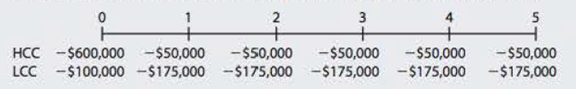Chapter 11, Problem 14P### Fundamentals of Financial Manageme...

9th Edition
Eugene F. Brigham + 1 other
ISBN: 9781305635937

#### Solutions

Chapter
Section### Fundamentals of Financial Manageme...

9th Edition
Eugene F. Brigham + 1 other
ISBN: 9781305635937
Textbook Problem
50 views

# CHOOSING MANDATORY PROJECTS ON THE BASIS OF LEAST COST Kim Inc. must install a new air conditioning unit in its main plant. Kim must install one or the other of the units; otherwise, the highly profitable plant would have to shut down. Two units are available, HCC and LCC (for high and low capital costs, respectively). HCC has a high capital cost but relatively low operating costs, while LCC has a low capital cost but higher operating costs because it uses more electricity. The costs of the units are shown here. Kim’s WACC is 7%.a. Which unit would you recommend? Explain. b. If Kim’s controller wanted to know the IRRs of the two projects, what would you tell him? c. If the WACC rose to 15% would this affect your recommendation? Explain your answer and the reason this result occurred.

a.

Summary Introduction

To explain: Whether the project HCC or LCC should be recommended on the basis of cost.

Introduction:

Capital Budgeting:

It refers to the long term investment decisions that has been taken by the top management of a company and that are irreversible in nature. These decisions require investment of large amount of the company’s cash.

Net Present Value (NPV):

It is a method under capital budgeting which includes the calculation of net present value of the project in which the company is investing. The calculation is done by calculating the difference between the value of cash inflow and value of cash outflow after considering the discounted rate.

Explanation

The calculation of NPV of project HCC on spreadsheet is:

Table (1)

The NPV for cost of the project HCC is -\$769,360.56.

The calculation of NPV of project LCC on spreadsheet is:

b.

Summary Introduction

To calculate: The IRR of the project HCC and LCC.

Introduction:

Internal Rate of Return (IRR):

It refers to the rate of return that is computed by the company to make a decision regarding the selection of a project for investment. This rate provides the basis for selection of projects with lower cost of capital and rejection of project with higher cost of capital.

c.

Summary Introduction

To explain: Whether project HCC or project LCC should be recommended at 15% WACC.

### Still sussing out bartleby?

Check out a sample textbook solution.

See a sample solution

#### The Solution to Your Study Problems

Bartleby provides explanations to thousands of textbook problems written by our experts, many with advanced degrees!

Get Started